## Tune Regression Model Using Experiment Manager

This example shows how to use Experiment Manager to optimize a machine learning regression model. The goal is to create a regression model for the `carbig` data set that has minimal cross-validation loss. Begin by using the Regression Learner app to train all available regression models on the training data. Then, improve the best model by exporting it to Experiment Manager.

In Experiment Manager, use the default settings to minimize the cross-validation loss (that is, minimize the cross-validation root mean squared error). Investigate options that help improve the loss, and perform more detailed experiments. For example, fix some hyperparameters at their best values, add useful hyperparameters to the model tuning process, adjust hyperparameter search ranges, adjust the training data, and customize the visualizations. The final result is a model with better test set performance.

For more information on when to export models from Regression Learner to Experiment Manager, see Export Model from Regression Learner to Experiment Manager.

1. In the MATLAB® Command Window, load the `carbig` data set, which contains measurements of cars made in the 1970s and early 1980s.

`load carbig`

The goal is to create a regression model that predicts the miles per gallon value for a car, based on the car's other measurements.

2. Categorize the cars based on whether they were made in the USA.

```Origin = categorical(cellstr(Origin)); Origin = mergecats(Origin,["France","Japan","Germany", ... "Sweden","Italy","England"],"NotUSA");```
3. Create a table containing the predictor variables `Acceleration`, `Displacement`, and so on, as well as the response variable `MPG` (miles per gallon).

```cars = table(Acceleration,Displacement,Horsepower, ... Model_Year,Origin,Weight,MPG);```
4. Remove rows of `cars` where the table has missing values.

`cars = rmmissing(cars);`
5. Partition the data into two sets. Use approximately 80% of the observations for model training in Regression Learner, and reserve 20% of the observations for a final test set. Use `cvpartition` to partition the data.

```rng("default") % For reproducibility c = cvpartition(height(cars),"Holdout",0.2); trainingIndices = training(c); testIndices = test(c); carsTrain = cars(trainingIndices,:); carsTest = cars(testIndices,:);```

### Train Models in Regression Learner

1. If you have Parallel Computing Toolbox™, the Regression Learner app can train models in parallel. Training models in parallel is typically faster than training models in series. If you do not have Parallel Computing Toolbox, skip to the next step.

Before opening the app, start a parallel pool of process workers by using the `parpool` function.

`parpool("Processes")`

By starting a parallel pool of process workers rather than thread workers, you ensure that Experiment Manager can use the same parallel pool later.

Note

Parallel computations with a thread pool are not supported in Experiment Manager.

2. Open Regression Learner. Click the Apps tab, and then click the arrow at the right of the Apps section to open the apps gallery. In the Machine Learning and Deep Learning group, click Regression Learner.

3. On the Learn tab, in the File section, click and select From Workspace.

4. In the New Session from Workspace dialog box, select the `carsTrain` table from the Data Set Variable list. The app selects the response and predictor variables. The default response variable is `MPG`.

5. In the Validation section, specify to use 3-fold cross-validation rather than the default 5-fold cross-validation.

6. In the Test section, click the check box to set aside a test data set. Specify `25` percent of the imported data as a test set.

7. To accept the options and continue, click .

8. Visually inspect the predictors in the open Response Plot. In the X-axis section, select each predictor from the X list. Note that some of the predictors, such as `Displacement`, `Horsepower`, and `Weight`, display similar trends.

9. Before training models, use principal component analysis (PCA) to reduce the dimensionality of the predictor space. PCA linearly transforms the numeric predictors to remove redundant dimensions. On the Learn tab, in the Options section, click .

In the Default PCA Options dialog box, click the check box to enable PCA. Select `Specify number of components` as the component reduction criterion, and specify `4` as the number of numeric components. Click .

10. To obtain the best model, train all preset models. On the Learn tab, in the Models section, click the arrow to open the gallery. In the Get Started group, click . In the Train section, click and select Train All. The app trains one of each preset model type, along with the default fine tree model, and displays the models in the Models pane.

11. To find the best result, sort the trained models based on the validation root mean squared error (RMSE). In the Models pane, open the Sort by list and select ```RMSE (Validation)```.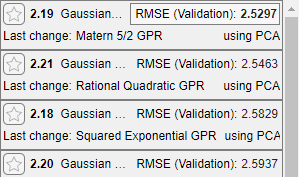Note

Validation introduces some randomness into the results. Your model validation results can vary from the results shown in this example.

### Assess Best Model Performance

1. For the model with the lowest RMSE, plot the predicted response versus the true response to see how well the regression model makes predictions for different response values. Select the Matern 5/2 GPR model in the Models pane. On the Learn tab, in the Plots and Results section, click the arrow to open the gallery, and then click in the Validation Results group.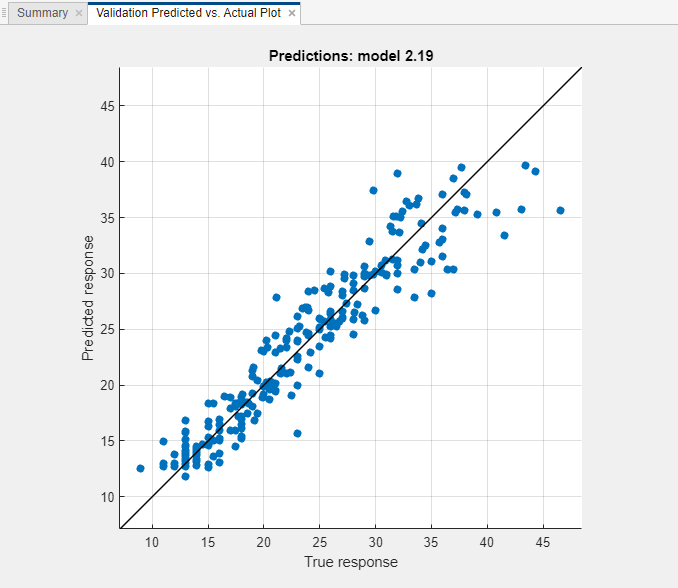Overall, the GPR (Gaussian process regression) model performs well. Most predictions are near the diagonal line.

2. View the residuals plot. On the Learn tab, in the Plots and Results section, click the arrow to open the gallery, and then click Residuals (Validation) in the Validation Results group. The residuals plot displays the difference between the true and predicted responses.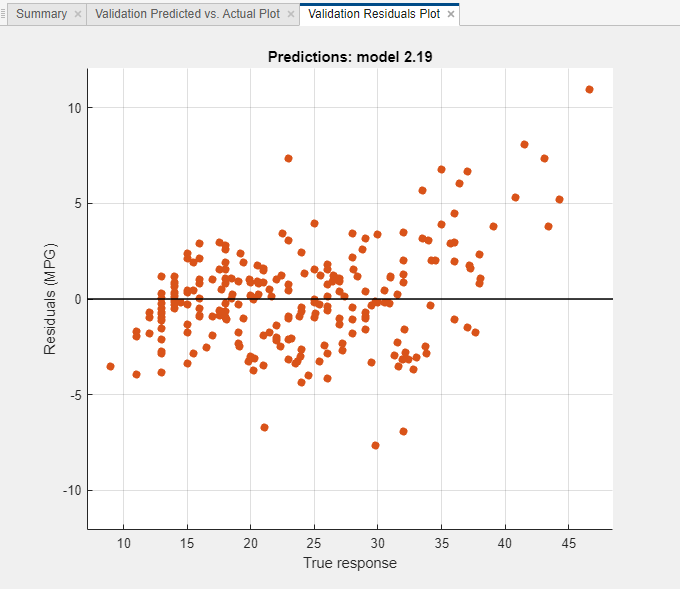The residuals are scattered roughly symmetrically around 0.

3. Check the test set performance of the model. On the Test tab, in the Test section, click Test Selected. The app computes the test set performance of the model trained on the full data set, including training and validation data.

4. Compare the validation and test RMSE for the model. On the model Summary tab, compare the RMSE (Validation) value under Training Results to the RMSE (Test) value under Test Results. In this example, the validation RMSE overestimates the performance of the model on the test set.### Export Model to Experiment Manager

1. To try to improve the predictive performance of the model, export it to Experiment Manager. On the Learn tab, in the Export section, click and select Create Experiment. The Create Experiment dialog box opens.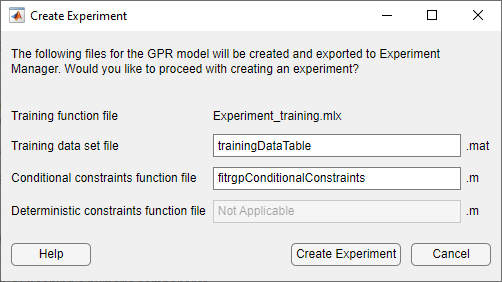2. In the Create Experiment dialog box, click . The app opens Experiment Manager and a new dialog box.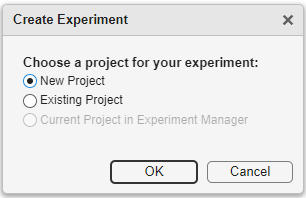3. In the dialog box, choose a new or existing project for your experiment. For this example, create a new project, and specify `TrainGPRModelProject` as the filename in the Specify Project Folder Name dialog box.

### Run Experiment with Default Hyperparameters

1. Run the experiment either sequentially or in parallel.

Note

• If you have Parallel Computing Toolbox, save time by running the experiment in parallel. On the Experiment Manager tab, in the Execution section, select `Simultaneous` from the Mode list.

• Otherwise, use the default Mode option of `Sequential`.

On the Experiment Manager tab, in the Run section, click .

Experiment Manager opens a new tab that displays the results of the experiment. At each trial, the app trains a model with a different combination of hyperparameter values, as specified in the Hyperparameters table in the Experiment1 tab.

2. After the app runs the experiment, check the results. In the table of results, click the arrow for the ValidationRMSE column and select Sort in Ascending Order.Notice that the app tunes the Sigma and Standardize hyperparameters by default.

3. Check the predicted vs. actual plot for the model with the lowest RMSE. On the Experiment Manager tab, in the Review Results section, click . In the Visualizations pane, the app displays the plot for the model.

To better see the plot, drag the Visualizations pane below the Experiment Browser pane.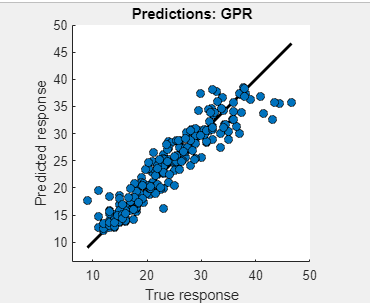For this model, the predicted values are close to the true response values. However, the model tends to underestimate the true response for values between 40 and 50 miles per gallon.

### Adjust Hyperparameters and Hyperparameter Values

1. Standardizing the numeric predictors before training seems best for this data set. To try to obtain a better model, specify the `Standardize` hyperparameter value as `true` and then rerun the experiment. Click the Experiment1 tab. In the Hyperparameters table, select the row for the `Standardize` hyperparameter. Then click .

2. Open the training function file. In the Training Function section, click . The app opens the `Experiment1_training1.mlx` file.

3. In the file, search for the lines of code that use the `fitrgp` function. This function is used to create GPR models. Standardize the predictor data by using a name-value argument. In this case, adjust the four calls to `fitrgp` by adding `'Standardize',true` as follows.

```regressionGP = fitrgp(predictors, response, ... paramNameValuePairs{:}, 'KernelParameters', kernelParameters, ... 'Standardize', true); ```
```regressionGP = fitrgp(predictors, response, ... paramNameValuePairs{:}, 'Standardize', true); ```
```regressionGP = fitrgp(trainingPredictors, trainingResponse, ... paramNameValuePairs{:}, 'KernelParameters', kernelParameters, ... 'Standardize', true); ```
```regressionGP = fitrgp(trainingPredictors, trainingResponse, ... paramNameValuePairs{:}, 'Standardize', true); ```

Save the code changes, and close the file.

4. On the Experiment Manager tab, in the Run section, click .

5. To further vary the models evaluated during the experiment, add hyperparameters to the model tuning process. In the MATLAB Command Window, use the `hyperparameters` function to see which hyperparameters you can tune for your model. Specify the training data set to see the default hyperparameter ranges. Enter the following code.

```load("trainingDataTable1.mat") info = hyperparameters("fitrgp",dataTable,"MPG"); for i = 1:length(info) disp(i);disp(info(i)) end```
``` 1 optimizableVariable with properties: Name: 'Sigma' Range: [1.0000e-04 78.9730] Type: 'real' Transform: 'log' Optimize: 1 2 optimizableVariable with properties: Name: 'BasisFunction' Range: {'constant' 'none' 'linear' 'pureQuadratic'} Type: 'categorical' Transform: 'none' Optimize: 0 3 optimizableVariable with properties: Name: 'KernelFunction' Range: {1×10 cell} Type: 'categorical' Transform: 'none' Optimize: 0 4 optimizableVariable with properties: Name: 'KernelScale' Range: [1.0000e-03 1000] Type: 'real' Transform: 'log' Optimize: 0 5 optimizableVariable with properties: Name: 'Standardize' Range: {'true' 'false'} Type: 'categorical' Transform: 'none' Optimize: 1```
6. Add the `BasisFunction`, `KernelFunction`, and `KernelScale` hyperparameters in Experiment Manager. For each hyperparameter, on the Experiment1 tab, in the Hyperparameters section, click . Edit the row entries to match the output of the `hyperparameters` function.

In particular, specify the `BasisFunction` range as `["constant","none","linear"]` and the `KernelFunction` range as `["ardexponential","ardmatern32","ardmatern52","ardrationalquadratic","ardsquaredexponential","exponential","matern32","matern52","rationalquadratic","squaredexponential"]`. Because the training data set includes a categorical predictor, omit the `pureQuadratic` value from the list of basis functions.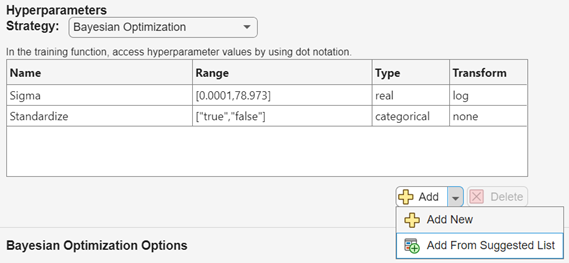For more information on the hyperparameters you can tune for your model, see Export Model from Regression Learner to Experiment Manager.

7. For better results when tuning several hyperparameters, increase the number of trials. On the Experiment1 tab, in the Bayesian Optimization Options section, specify `60` as the maximum number of trials.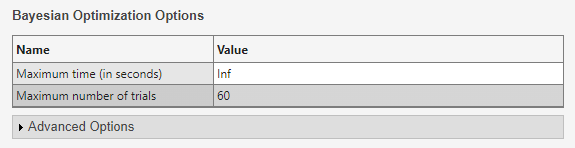8. On the Experiment Manager tab, in the Run section, click .

### Specify Training Data

1. Before running the experiment again, specify to use all the observations in `carsTrain`. Because you reserved some observations for testing when you imported the training data into Regression Learner, all experiments so far have used only 75% of the observations in the `carsTrain` data set.

Save the `carsTrain` data set as the file `fullTrainingData.mat` in the `TrainGPRModelProject` folder, which contains the experiment files. To do so, right-click the `carsTrain` variable name in the MATLAB workspace, and click Save As. In the dialog box, specify the filename and location, and then click .

2. On the Experiment1 tab, in the Training Function section, click .

3. In the `Experiment1_training1.mlx` file, search for the `load` command. Specify to use the full `carsTrain` data set for model training by adjusting the code as follows.

```% Load training data fileData = load("fullTrainingData.mat"); trainingData = fileData.carsTrain;```
4. On the Experiment1 tab, in the Description section, change the number of observations to `314`, which is the number of rows in the `carsTrain` table.

5. On the Experiment Manager tab, in the Run section, click .

1. You can add visualizations for Experiment Manager to return at each trial. In this case, specify to create a residuals plot. On the Experiment1 tab, in the Training Function section, click .

2. In the `Experiment1_training1.mlx` file, search for the `plot` function. The surrounding code creates the validation predicted vs. actual plot for each trained model. Enter the following code to create a residuals plot. Ensure that the residuals plot code is within the `trainRegressionModel` function definition.

```% Create validation residuals plot residuals = validationResponse - validationPredictions; f = figure("Name","Residuals (Validation)"); resAxes = axes(f); hold(resAxes,"on") plot(resAxes,validationResponse,residuals,"ko", ... "MarkerFaceColor","#D95319") yline(resAxes,0) xlabel(resAxes,"True response") ylabel(resAxes,"Residuals (MPG)") title(resAxes,"Predictions: GPR")```
3. On the Experiment Manager tab, in the Run section, click .

4. In the table of results, click the arrow for the ValidationRMSE column and select Sort in Ascending Order.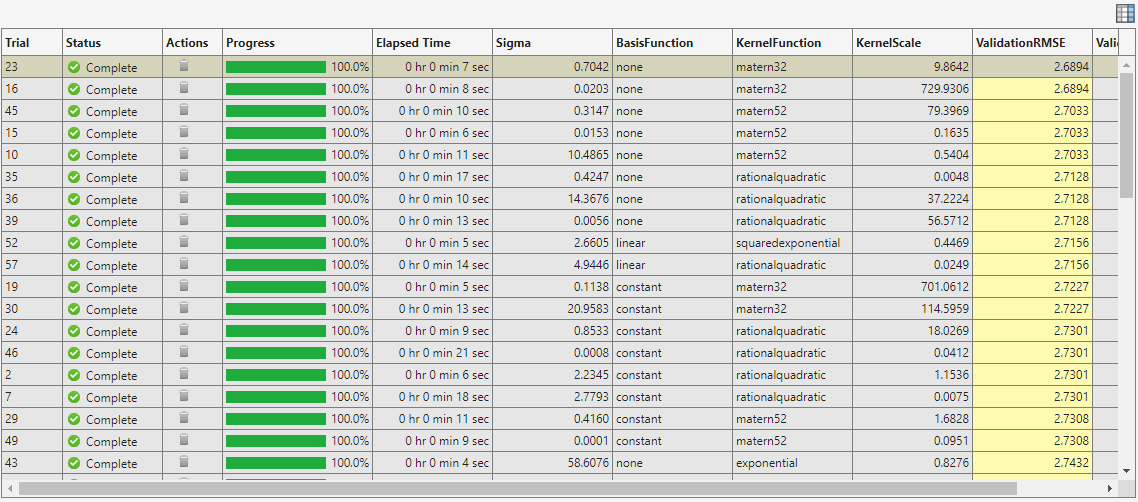5. Check the predicted vs. actual plot and the residuals plot for the model with the lowest RMSE. On the Experiment Manager tab, in the Review Results section, click . In the Visualizations pane, the app displays the plot for the model.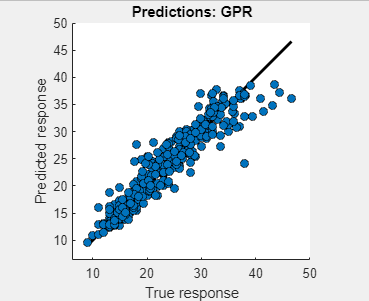6. On the Experiment Manager tab, in the Review Results section, click . In the Visualizations pane, the app displays the plot for the model.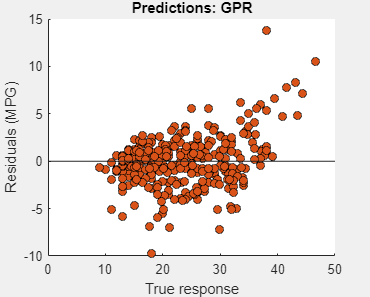Both plots indicate that the model generally performs well.

### Export and Use Final Model

1. You can export a model trained in Experiment Manager to the MATLAB workspace. Select the best-performing model from the most recently run experiment. On the Experiment Manager tab, in the Export section, click and select Training Output.

2. In the Export dialog box, change the workspace variable name to `finalGPRModel` and click .

The new variable appears in your workspace.

3. Use the exported `finalGPRModel` structure to make predictions using new data. You can use the structure in the same way that you use any trained model exported from the Regression Learner app. For more information, see Make Predictions for New Data Using Exported Model.

In this case, predict the response values for the test data in `carsTest`.

`testPredictedY = finalGPRModel.predictFcn(carsTest);`
4. Compute the test RMSE using the predicted response values.

```testRSME = sqrt((1/length(testPredictedY))* ... sum((carsTest.MPG - testPredictedY).^2))```
```testRSME = 2.6647```

The test RMSE is close to the validation RMSE computed in Experiment Manager (`2.6894`). Also, the test RMSE for this tuned model is smaller than the test RMSE for the Matern 5/2 GPR model in Regression Learner (`3.0267`). However, keep in mind that the tuned model uses observations in `carsTest` as test data and the Regression Learner model uses a subset of the observations in `carsTrain` as test data.

5. Create a predicted vs. actual plot and a residuals plot using the true test data response and the predicted response.

```figure line([min(carsTest.MPG) max(carsTest.MPG)], ... [min(carsTest.MPG) max(carsTest.MPG)], ..., "Color","black","LineWidth",2); hold on plot(carsTest.MPG,testPredictedY,"ko", ... "MarkerFaceColor","#0072BD"); hold off xlabel("True response") ylabel("Predicted response")```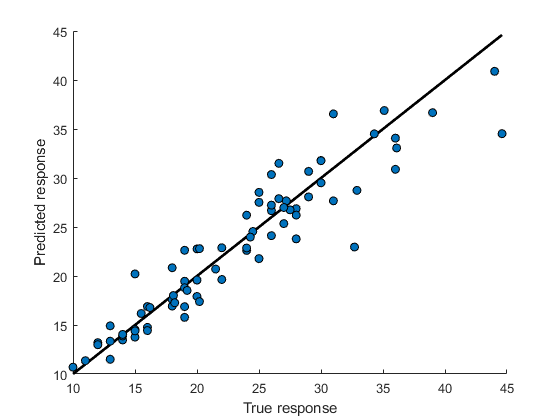```figure residuals = carsTest.MPG - testPredictedY; plot(carsTest.MPG,residuals,"ko", ... "MarkerFaceColor","#D95319") hold on yline(0) hold off xlabel("True response") ylabel("Residuals (MPG)")```Both plots indicate that the model performs well on the test set.